# Python Correlation – A Practical Guide

## What is correlation?

A correlation is a relationship between two sets of data.

In the equity markets, for example, you may notice that stocks like Microsoft (MSFT) and Apple (AAPL) both tend to rise and fall at the same time. The price behavior between the two stocks is not an exact match, but there is enough similarity to say there is a relationship. In this scenario, we can say MSFT and AAPL have a positive correlation.

Further, there are often relationships across markets. For example, between equities and bonds, or precious metals. We often also see a correlation between financial instruments and economic data or even sentiment indicators.

## Why do correlations matter?

There are several reasons why correlations are important, here a few benefits of tracking them in the markets –

1. Insights – keeping track of different relationships can provide insight into where the markets are headed. A good example is when the markets turned sharply lower in late February as a result of the Coronavirus escalation. The price of gold, which is known as an asset investors turn to when their mood for risky investments sours, rose sharply the trading day before the big initial drop in stocks. It acted as a warning signal for those equity traders mindful of the inverse correlation between the two.
2. Strength in correlated moves – It’s much easier to assess trends when there is a correlated move. In other words, if a bulk of the tech stocks on your watchlist are rising, it’s probably safe to say the sector is bullish, or that there is strong demand.
3. Diversification – To make sure you have some diversification in your portfolio, it’s a good idea to make sure the assets within it aren’t all strongly correlated to each other.
4. Signal confirmation – Let’s say you want to buy a stock because your analysis shows that it is bullish. You could analyze another stock with a positive correlation to make sure it provides a similar signal.

## Correlation doesn’t imply causation

A popular saying among the statistics crowd is “correlation does not imply causation”. It comes up often and it’s important to understand its meaning.

Essentially, correlations can provide valuable insights but you’re bound to come across situations that might imply a correlation where a relationship does not exist.

As an example, data has shown a sharp rise in Netflix subscribers as a result of the lockdown that followed the Coronavirus escalation. The idea is that people are forced to stay at home and therefore are more likely to watch tv.

The same scenario has resulted in a rise in electricity bills. People are using more electricity at home compared to when they were at work all day.

If you were blindly comparing the rise in Netflix subscribers versus the rise in electricity usage during the month of lockdown, you might reasonably conclude that the two have a relationship.

However, having some perspective on the manner, it is clear that the two are not related and that it is not likely that fluctuations in one will impact the other moving forward. Rather, it is the lockdown, an external variable, that is the causation for both of these trends.

## What is a correlation coefficient?

We’ve discussed that fluctuations in the stock prices of Apple and Microsoft tend to have a relationship. You might then notice other tech companies also correlate well with the two.

But not all relationships are equal and the correlation coefficient can help in assessing the strength of a correlation.

There are a few different ways of calculating a correlation coefficient but the most popular methods result in a number between -1 and +1.

The closer the number is to +1, the stronger the relationship. If the figure is close to -1, it indicates that there is a strong inverse relationship.

In the finance world, an inverse relationship is where one asset rises while the other drops. As one of the previous examples suggested, stocks and the price of gold have a long-standing inverse relationship.

The closer the correlation coefficient is to zero, the more likely it is that the two variables being compared don’t have any relationship to each other.

## Breaking down the math to calculate the correlation coefficient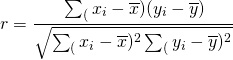The above formula is what’s used to calculate a correlation coefficient using the Pearson method.

It might look a bit intimidating the first time you look at it (unless you’re a math pro ofcourse). But we will break down this formula and at the end of it you will see that it is just basic mathematics.

There are libraries available that can do this automatically, but the following example will show how we can make the calculation manually.

We will start by creating a dataset. We can use the Numpy library to create some random data for us. Here is the code:

import numpy as np
import pandas as pd
df = pd.DataFrame(np.random.randint(0,10,size=(5, 2)), columns=list('xy'))

The image below shows what my DataFrame looks like. If you’re following along, the data will look different for you as Numpy is filling in random numbers. But the format should look the same.

Now that we have a dataset, let’s move on to the formula. We will start by separating out the first part of the formula.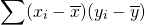We can break this down further. Remember BODMAS? it states that we must perform what is in the brackets first.For the formula above, we need to take each value of x and subtract it by the mean of x.

We can use the mean() function in Pandas to create the mean for us. Like this:

df.x.mean()

But we still need to subtract the mean from x. And we also need to temporarily store this information somewhere. Let’s create a new column for that and call it step1.

df['step1'] = df.x - df.x.mean()

This is what our DataFrame looks like at this point.

Now that we have the calculations needed for the first step. Let’s keep going.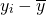The second step involves doing the same thing for the y column.

df['step2'] = df.y - df.y.mean()

That’s easy enough, what’s next?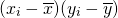The formula is telling us that we need to take all the values we gathered in step 1 and multiply them by the values in step 2. We will store this in a new column labeled step3.

df['step3'] = df.step1 * df.step2

This is what the DataFrame looks like at this point:

We can now move on to the last operation in this part of the formula.if you’re not familiar with this symbol: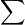It stands for sum. This means we need to add up all the values from the previous step.

step4 = df.step3.sum()

Great, we have summed up the values and have stored it in a variable called step4. We will come back to this later. For now, we can start on the second part of the formula.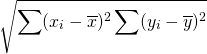Let’s follow the same steps and break down the formula.Does this look familiar? we have already done this in step 1 so we can just use that data.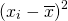The next part of the formula tells us we have to square the results from step 1. We will store this data in a new column labeled step5.

df['step5'] = df.step1 ** 2

The next part of the formula tells us to do the same thing for the y values.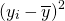We can take the values that we created in step 2 and square them.

df['step6'] = df.step2 ** 2

This is what our DataFrame looks like at this point:

Let’s look at the next part of the formula: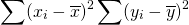This tells us that we have to take the sum of what we did in step 5 and multiply it with the sum of what we did in step 6.

step7 = df.step5.sum() * df.step6.sum()

Let’s keep going, almost there!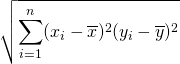The last portion of this part is to simply take the square root of the figure from our previous step. We can use the Numpy library to calculate the square root.

step8 = np.sqrt(step7)

Now that we’ve done that, all that is left is to take the answer from the first part of the formula and divide it by the answer in the second part.

step4/step8

And there you have it, we’ve manually calculated a correlation coefficient. To make sure that the calculation is correct, we can will use the corr() function which is built into Pandas to calculate the coefficient.

df.x.corr(df.y)

Here is our final result. Your correlation coefficient will be different, but it should match the output from the Pandas calculation.

## How can I calculate the correlation coefficients for my watchlist in Python?

Calculating a correlation coefficient in Python is quite simple as there are several libraries that can do the heavy lifting for you.

The code for all of the examples in this guide are available on GitHub if you’re interested in following along. In addition to the Python files a Jupyter notebook version is also available.

### Step one – Gathering and cleaning up historical data

This step can be painfully time-consuming, however, we recently published a guide on how to download data from Alpha Vantage using their free API. This allows us to obtain our data, pre-formatted, in just a few lines of code.

We are using the Alpha Vantage library in this step so if you are not familiar, we recommend having a read through the guide as there are some important steps such as storing your API keys as environment variables.

import pandas as pd
from alpha_vantage.timeseries import TimeSeries

Our first step is to import the Pandas library as we will be using it to store out data as well as calculating the correlation coefficient. We’ve also imported the Timeseries class from the alpha_vantage library which will be used to retrieve historical data.

We have exported our watchlist to a CSV file so in the next step we will import it and convert it to a list format. There are several ways to read a CSV file in Python but since we are already using Pandas, we might as well use it here rather than importing another library just for this step.

If you don’t have your watchlist in CSV format, you can just as easily create a Python list that includes the tickers within your watchlist.

#grab tickers from csv file
watchlist = watchlist_df.iloc.tolist()

We now have a Python list of the five stock tickers that we will be using in this example. Our next step is to itter through the watchlist and download historical data.

#instantiate TimeSeries class from alpha_vantage library
app = TimeSeries(output_format='pandas')

First, we instantiate the Timeseries class from the alpha_vantage library. We’ve passed through a parameter here so that the output will be a Pandas dataframe. This will save a lot of time having to format the data.

#itter through watchlist and retrieve daily price data
stocks_df = pd.DataFrame()
for ticker in watchlist:
alphav_df = alphav_df
alphav_df.columns = [i.split(' ') for i in alphav_df.columns]

stocks_df[ticker] = alphav_df['adjusted'].pct_change()

Next, we itter through our Python list of stock tickers and call the Alpha Vantage API for data for each ticker. But before doing that, we’ll create an empty Pandas dataframe that we can append data to.

What we’ve done is taken the ‘adjusted’ column, which is the adjusted daily close, and appended it to our stocks_df dataframe. Note the additional pct_change() function. This will normalize our data by converting the price data to a percentage return. We will talk about the reason behind this in more detail further in the guide. This is what our dataframe looks like at this point.

How sweet is that! a nicely formated time-series dataframe in less than 20 lines of code!

### Step Two – Calculating the correlation coefficient

Now that we have our data, we can easily check the correlation coefficient between any of the stocks within our dataframe. Here is how we check the correlation between AAPL and MSFT.

print(stocks_df.AAPL.corr(stocks_df.MSFT))

What we’ve done here is taken the column of adjusted closing prices for AAPL and compared it with the column for MSFT. To access a single column, we specify the name of the dataframe and column like so:

print(stocks_df.AAPL)

alternatively, we can also access it like this:

print(stocks_df['AAPL'])

When dealing with a single column we are no longer working with a dataframe. Rather, we are working with a Pandas series. The basic syntax for calculating the correlation between different series is as follows:

Series.corr(other_series)

In our example, we found a correlation coefficient of 0.682 between AAPL and MSFT. Remember, the closer to 1, the higher the positive correlation. So in this example, there is a very strong correlation between these two stocks.

Let’s take a look at the correlation between Apple and Netflix:

print(stocks_df.AAPL.corr(stocks_df.NFLX)

The correlation coefficient is -0.152. It’s quite close to zero which indicates that there was no correlation between these two stocks. At least, during that time period.

There are three main methods used in calculating the correlation coefficient: Pearson, Spearman, and Kendall. We will discuss these methods in a bit more detail later on in the guide.

By default, Pandas will use the Pearson method. You can pass through different methods as parameters if you desire to do so. Here is an example of a calculation using the Spearman method:

print(stocks_df.AAPL.corr(stocks_df.NFLX, method='spearman')

And this is how you would get the correlation coefficient using the Kendall method:

print(stocks_df.AAPL.corr(stocks_df.NFLX, method='kendall'))

## Correlation of returns versus prices

We calculated the percentage return between each price point in our dataset and ran our correlation function on that rather than calculating it on the raw data itself. We do this to get a more accurate correlation coefficient.

The reasoning behind it is that it standardizes the data which is beneficial no matter which calculation method you use.

If you’re using the Spearman or Kendall method, which utilize a ranking system, returns data will remove some of the extremes from your dataset which can otherwise influence the entire ranking system.

The Pearson method doesn’t use a ranking system but heavily relies on the mean of your data set. Using returns data narrows the range of your dataset which in turn puts more emphasis on deviations from the mean, resulting in a higher accuracy.

values_x = [10, 11, 13, 16, 17, 4, 5, 6]
values_y = [10, 11, 13, 16, 17, 18, 19, 20]

Take a look at the above two datasets as an example.

Notice how they both have almost the same data? The difference is that values_x dropped off sharply in the third last value from 17 to 4. However, it continued to rise by one in the last two values, the same way values_y did.

This type of behavior can happen often in the markets. For example, a stock might have reported earnings which caused a sharp but temporary drop in its price. But aside from the momentary drop, the overall fluctuations in the stock price have not changed much at all compared to other correlated stocks.

The ranking systems used in correlation calculations, however, will view the momentary decline differently. It will assign an arbitrarily low value to the last three values in values_x since they are the lowest in the dataset. At the same time, it will rank the last three values in values_y as the largest.

This creates a major discrepancy that will ultimately cause our correlation coefficient to be much lower than it should be.

In a non-ranking system such as the Pearson method, the last three values will drag down the mean value for the entire dataset.

If we take the returns instead, we are comparing how much one value fluctuated relative to the value before it.

In that case, there would have been a major decline when the values in values_x dropped from 17 to 4, but the divergence in correlation stops there as both the data sets rose in value in the last two places.

## How can I create a time-series dataset in Pandas?

A time-series is simply a dataset that follows regular, timed intervals. The previous example, where we had data for five stocks, is a good example of a time-series dataset.

Further, Pandas intuitively lined up price data when we merged all five stocks into one dataframe, based on the date column which all of our data had in common. This column then acts as an index for our data.

We can just as easily create a dataframe with a time-series index from scratch. The next example will show how to do that with data we have saved in a CSV file.

import pandas as pd

print(TSLA_df)

Here we’ve imported price data for TSLA based on 15-minute intervals. In other words, 15-minute bars for TSLA.

Notice that Pandas has created a generic index rather than using the date column. We can change that with two methods. Either we manually set the index like this:

TSLA_df.set_index('date', inplace=True)

Or, we can pass in a parameter in the prior function where we imported the data.

TSLA_df = pd.read_csv('TSLA.CSV', index_col=0)

This will tell Pandas to use the first column in the CSV data as the index which in price data will typically be your date or time data.

Next we will check the data type for our newly-created index.

print(TSLA_df.index[:4])

As you can see, the dtype shows the index as an object. We can convert it to a DateTime like so:

TSLA_df.index = pd.to_datetime(TSLA_df.index)

If we check the index again, we will now see the dtype as ‘datetime64[ns]’ which is what we are after.

When importing a CSV file, we can pass through parse_dates=True into the pd.read_csv() function to automatically parse the dates as a DateTime object.

TSLA_df = pd.read_csv('TSLA.CSV', index_col=0, parse_dates=True)

We did it manually in this example just to illustrate how it can be done in the event you are creating a dataframe using other methods than from a CSV.

## What is a correlation matrix?

The previous examples have shown how to calculate a correlation coefficient for two stocks. But if we have a dataframe full of stocks? surely there has to be an easier way to get the coefficient for everything in the dataframe?

That’s where the correlation matrix comes in. It is a table, or a matrix rather, that will display the correlation coefficient for everything in the dataframe. To create this simply type your dataframe name followed by .corr(). Or in our example, stocks_df.corr().

Here we have our correlation matrix. The very first column in the first row is the correlation between AAPL and AAPL which obviously, when comparing data with itself, will have the highest correlation.

Looking at this matrix, we can easily see that the correlation between Apple (AAPL) and Exxon Mobile (XOM) is the strongest while the correlation between Netflix (NFLX) and AAPL is the weakest.

Further, there is fairly notable negative correlation between AAPL and GLD which is an ETF that tracks gold prices.

We can also create a heatmap. This will allow us to visualize the correlation between the different stocks.

To do this, we will use the Seaborn library which is a great tool for plotting and charting. It is built on top of the popular matplotlib library and does all the heavy lifting involved in creating a plot.

import seaborn as sns
import matplotlib.pyplot as plt

ax = sns.heatmap(stocks_df.corr())
plt.show()

Here we’ve imported the library and called the heatmap function to display the heatmap. At this stage, we’ve only passed through the correlation matrix dataframe.

We can now asses the strength in correlation based on color and there is a useful guide on the right-hand side. But since we are used to seeing things in red and green in the finance world, let’s customize it a bit.

ax = sns.heatmap(stocks_df.corr(), cmap='RdYlGn', linewidths=.1)
plt.show()

The above code snippet sets the Red, Yellow, Green values to cmap which defines our colors. We have also passed through a line width of .1 to create a bit of space between the boxes just to improve the visual aesthetics.

There you have it. It is much easier to see that AAPL and NFLX have the weakest correlation. We can also easily see that GLD has a negative correlation with all of the other assets.

## How to use a correlation matrix in practice?

You can use a correlation matrix to quickly filter out stocks for various reasons. Maybe you’re already in a trade and you don’t want to trade other instruments with a strong correlation. Another reason might be to check other strongly correlated instruments to ensure you’re analysis is producing a similar signal.

As an example, say you’ve already taken a long position in AAPL. Now your automated trading algo is sending you a signal to buy MSFT. This is very likely to happen since we’ve already determined that the two have a strong correlation with each other.

In this case, you might want to skip that trade because it is only increasing your risk exposure. In other words, when the correlation is that high, it’s not all that different from just doubling up your exposure in AAPL, and that is something to avoid.

In the same way, we can also confirm if our signal is strong enough to act on. For example, let’s say we are trading a breakout strategy and we buy a stock when it exceeds more than one standard deviation from its average.

We get a signal to buy NFLX. We can see what stock is most closely correlated with NFLX to determine if it has also exceeded one standard deviation from its average. We can use the idxmax() function from Pandas to figure out the strongest correlation.

nflx_corr_df = stocks_df.corr().NFLX
print(nflx_corr_df.idxmax())

But wait, we already know that the highest correlation is going to be with NFLX itself, it produces a correlation of 1. So we want to filter for correlations less than 1.

print(nflx_corr_df[ nflx_corr_df < 1 ].idxmax())

The above code returns ‘MSFT’. Now we can check where Microsoft is trading relative to its standard deviation. If it is trading below it, we can even wait until it exceeds it to give us a stronger signal on our original NFLX buy signal.

In the same manner, we can easily check for inverse correlations with NFLX as follows

print(nflx_corr_df.idxmin())

This returned ‘XOM’. If our analysts gives us a bearish signal for XOM it would once again provide more conviction on our bullish NFLX trade.

## What are some of the different libraries in Python used for correlation?

We have focused a lot on Pandas but there are several libraries available that can be used to calculate the correlation coefficient and other statistical measures.

To provide a bit of background, Pandas is part of the SciPy stack. Two different libraries within this stack can be used for correlation calculations – NumPy and SciPy.

Pandas is built on top of NumPy, maximizing the concept of 3D arrays. NumPy is known for its speed and its ability to create multi-dimensional arrays and matrices. It is the backbone for several financial libraries and without it, Python probably would have never gained the popularity it has now within the financial community.

The downside is that it has a slightly larger learning curve which is why Pandas is more commonly used in financial applications.

The upside of NumPy is that it is largely written in C which offers a speed benefit.

## What is the difference between covariance and correlation?

Both aim to provide insight into the relationship between two datasets. The simple difference between the two is that covariance does not provide any information on the strength of the relationship.

Covariance will simply tell you if there is a positive or negative relationship based on if the covariance is positive or negative.

Further, while a correlation coefficient has a standard range between -1 and +1, covariance does not have a range and theoretically, values can vary from –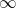to +.

We can create a covariance matrix with the following code – stocks_df.cov()

The table above shows the covariance among the stocks. Note that the covariance when comparing stocks with themselves have no consistency.

Also, we have no way of telling which correlation are the strongest or weakest. The table simply tells us if the stocks in it are positively or negatively correlated with each other.

## What is the difference between correlation and regression analysis?

The two have a lot of similarities but are distinctly different in their purpose. A correlation coefficient is used in the calculation of regression analysis just to give you an idea of how close the two are related.

Regression analysis is more commonly used for prediction. More specifically, it is used to predict the value of x based on the value of y. In contrast, the correlation coefficient aims at defining the relationship between x and y.

Here are some examples where you might look to use regression rather than correlation

• Predicting changes in currency or precious metals based on interest rate changes
• Mean reversion in a basket of strongly correlated instruments
• Projecting stock fluctuations based on economic reports or earnings data.

The main thing to keep in mind is that with regression analysis you usually have two sets of data. You have your independent values and your dependent values. In other words, you have value x, which will be used to predict value y, which you may not have yet. In contrast, correlation is more often used when both values are available.

### Do I need to use both correlation and regression analysis?

It depends on what you are trying to do, but generally speaking, it is a good idea to use correlation when you are using regression analysis.

As mentioned, regression analysis utilizes the correlation coefficient in its formula. More specifically, it uses the square of the correlation coefficient, otherwise known as R squared.

Let’s back up for a moment. We’ve done some examples that show how to get the correlation coefficient. This is also known as R. So what is R squared?

R squared is simply a method that makes it easier to assess the strength of the correlation compared to other correlations. For example, if you have an R squared of 0.7 and 0.5 you can definitively say that the former is 1.4 times as good as the latter. With just R, you can determine whether one correlation is better than the other, but you cannot define exactly by how much.

So it’s a good idea the check the strength of correlation in regression analysis although some of that information can be had just by looking at r squared.

### Can I just rely on the R-squared of the regression analysis to determine correlation?

In regression analysis, R squared is used so that negative deviations from the mean are not excluded when we sum them. By taking the square of R, it is always a positive value.

This presents a problem if you’re using the R squared from your regression analysis to asses just plain correlation. As the figure will always be positive, there is no way to determine if the correlation is positive or negative.

So while it can tell you about the strength of the correlation, it is a good idea to calculate to correlation coefficient separately to determine if the correlation is positive or negative.

## Which correlation method should I use – Pearson, Kendall, or Spearman?

The simple answer here is that you will probably use the Pearson method in financial applications.

The big difference between the three is that the Spearman and Kendall methods use a ranking system where the Pearson method does not.

This means, you can use the Spearman or Kendall methods on qualitative data. In other words, data that can’t be measured quantitatively but can still be ranked.

As an example, let’s say you’re measuring the correlation of social media sentiment against the performance of the stock market. However, your social media data will only indicate whether sentiment is bullish, very bullish, bearish or very bearish.

The social media represents qualitative data. It is not in numerical form yet we can still measure or rank the data. For this reason, a ranking method like Spearman or Kendall works best.

Another important factor is the distribution of your data. The Pearson method is used for linear data such as a time-series. For a non-linear dataset, the Spearman or Kendall method can be used. Non-linear is simply when there isn’t a regular interval for your data.

When it comes to the difference between Spearman and Kendall, the latter tends to have a more accurate result in smaller datasets. So if your data is limited, Kendall’s Tao is the way to go.

Lastly, regression analysis is only performed on linear data and it relies on the Pearson method in determining it’s correlation coefficient, or R. For the sake of consistency, this is another reason why it’s a good idea to use the Pearson method.

## How do you spot and avoid spurious correlations?

In the previous section, correlation doesn’t imply causation, we discussed an example of where two sets of data can seem to have a relationship when in fact they do not.

In that particular example, the two sets of data were driven by the same causality. But there are cases where two sets of data seem to have a relationship by pure coincidence.

In either case, the lack of a real relationship between data where it seems like there is one is known as a spurious correlation.

There is a book written on spurious correlations by Tyler Vigen who also has a website that comically illustrates seemingly correlated data where a relationship is clearly not present.

As an example, he displays on his website the relationship between the number of people that have drowned by falling into a pool versus the number of films Nicolas Cage has appeared in. It had a shocking 66.6% correlation! Obviously the two don’t have any relationship whatsoever.

In finance, we can avoid spurious correlations by checking for correlations over longer periods.

That doesn’t mean that two sets of data have to correlate for several years for it to be a valid correlation. For example, you might see a correlation between bonds and the US dollar for a few weeks, and then it disappears only to come back a month later. If this type of behavior continues for an extended period, there is a good chance it is not a spurious correlation.

Sometimes, you might have to dig a bit deeper to determine if a correlation is spurious in the financial markets. This could involve researching fundamental drivers and the impact said drivers had in the past.

## What is lagging correlation?

A lagging correlation is where two sets of data carry a correlation although the distribution might vary. As an example, you might find that one stock follows another, however, it does so an hour later or maybe even a day later.

These types of correlations are not all that easy to find, but when you do you might feel like you’ve hit the jackpot. They can produce good profits in the markets if you can find persistent lagging correlations with a high R or R squared.

One reason why you might find such a correlation is that the markets have shown an increasingly stronger correlation across assets over the years as a result of a rise in machine trading.

Some automated systems look for lagging assets which could cause a lagging correlation in price movement. For example, let’s say all the bank stocks are rallying yet there is one stock that is falling behind. At some point, an automated system will perceive value and look to buy that stock which triggers a delayed reaction.

You may also notice that when the market is just starting to gain strength, a few select stocks will break to new highs first. The same is true during bear markets, the weakest stocks often turn lower weeks if not months before the broader markets do.

## How to use lagging correlation in practice?

There is a function within Pandas that allows you to ‘shift’ your data up or down. Here is an example.

The image above is a printout of the first five rows from our dataframe that contains daily closing prices for the S&P 500 (SPY). Let’s see what happens when we call the shift() command which is a built-in function of the Pandas library.

As you can see, the dates have remained unchanged but daily price data has ‘shifted’ down one row. The first row now reads NaN, or Not a Number, as a result.

We can just as easily shift the values up like so:

Next, we will look at how we can find lagging correlations using the shift function within Pandas.

We have downloaded a CSV that contains two sets of values. The first is the spread between the 10-year and 2-year bonds. The second is the daily closing price for the S&P 500 (SPY).

Let’s start by creating a time-series dataframe from our CSV using the methods from earlier examples.

import pandas as pd

df.index = pd.to_datetime(df.index, unit='s')

Our data goes back 10 years but we only want to take a small sample size. It is rare for lag correlations to last a long time so we will focus on a much smaller period.

march = df['2020-03']
march_high = march.close.idxmax()

What we’ve done in the code snippet above is creat a new dataframe called March which will contain only data from March.

We then took the index value for the highest closing price of the bond spread from our new March dataframe. This will be our starting point for measuring the correlation coefficient.

bonds = march[march_high:].close.pct_change()
spy = march[march_high:].SPY.pct_change()

We then split the dataframe into two separate Pandas series. This step is not necessary but makes the code a bit easier to read.

While splitting the dataframe, we’ve also used the pct_change() function to normalize our data.

We can now check the correlation between the two.

print(bonds.corr(spy))

The code above returned a value of 0.45. This suggests that the two have a somewhat weak positive correlation.

Let’s see what happens if we shift the data.

print(bonds.corr(spy.shift(-2)))

Our correlation is now at -0.62. This is a much stronger correlation. More importantly, it is a negative correlation which tells us something completely different from what our initial figure told us.

Now that we know that SPY is lagging 2 days behind the bond spread, we can use the price movements in the bond spread as a guide as to where SPY might go next.

This process may seem easy in our example but we spend a bit of time looking at these instruments on a charting platform to determine the ideal shift ahead of time. In reality, this type of analysis often requires a lot of experimenting and trial and error before figuring out the exact lag in correlation.

It’s important to remember that these correlations come and go. Just because we’ve discovered a lag correlation doesn’t mean the relationship will continue.

Also, going back to the point about spurious correlations, it is a good idea to go through the data and try and spot several different periods where SPY and the bond spread had a lag correlation. This will help to validate whether the relationship is a spurious correlation or not.

The code snippets used in the examples are available on GitHub. From the GitHub page, click the green button on the right “Clone or download” to download or clone the code.

113 Views